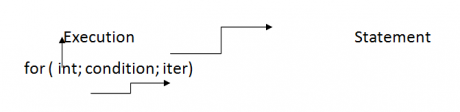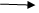Different types of loops in C
Ratings:
(4.2)
Views:2905## About Different types of loops in C Language

In C programming language we are having 3 types of loops

3. #### do- while

1. While loop

When we are work-in with while loop then always pre checking process is ocuur i.e. before execution of the statement black condition will be executed. while loop will repeats in clock direction. syntax: Assignment; while(condition) { statement1; statement2; statement3; . . . . . . .  . .. . . . . . . . inc/dec; }

• #### Void main()

{ int I; i=1; while(i<=10) { printf(“%d”,i); ++I; } }

## For loop:

When we are working with for loop it contains 3 part i.e.

1. Initialization
2. Condition
3. Iteration

### Syntax:

for (initialisation ; condition ; iteration) { statement block; }Control passing for condition when we are working with for loop always execution process will starts from intialisation block. initialization block will be executed only once whom we are passing the control within the body first time. After execution of the initialization part control will pass to iteration, from iteration part once again it will pass to condition Always repetition will happen b/w condition statement block and iterations only. When we are working with for loop everything is optional but mandatory to place 2 semicolons while() error for(; ;) yes valid   when the condition part is not available in for loop it repeats infinite times because condition part is replaced with non-zero value. when we are working with for loop pre checking process will be occur i.e before execution if the body condition part will executed. for loop will repeats in anti clock direction   while(0)No repetition for(;0;)is it will repeat.   When for loop condition part is replaced within constant ‘0’ then it repeats once because at the time of complilation number of instances became one int I; i=0; while(i)no repetition for (., I, )no repetition

• ### void main()

{ inti; for( i=1 ; i<=10; i++) printf(“%d”,i); } O/p: 1 2 3 4 5 ……..9 10

## Do while:

In implementation when we required repeating the statement block at least once then go for do-while. Indo-while loop post checking process will be occur i.e. after execution of the body, condition part will be executed. Do while loop also will repeats in clock direction Syntax : Assignment do { statement1; statement2; ….. … ……. includes; } while (condition ); According to the syntax of the do –while semi colon(;) must be required a end of the while.

• ### Void main()

{ int I; i=1; do { printf(“%d”,i); ++i; } while (i<=10) O/P: 1 2 3 …………… 8  9 10

• ### Void main()

{ int a a=2; for a(  ; a<=20; ) { printf (“%d”, a); a+=2; } } O/p : 2 4  6 ……….. 18 20 Advantage : for loop is faster than while loop.

### Void main()

{ int a,b; for(a=1;b=10;a<=b;a++,b--) printf(“\n %d %d ,a,d) printf (“\n a=%d d=%d ,a+10,a+10); } O/p:         1        10 2        9 3        8 4        7 5        6 a=16 b=15 for(a=1;b=10;a<=b;a++,b--_ i<=10 pf(“%d%d”, a,b); pf(“%d %d”, a+10,b+10) 6+10,5+10   In implementation when we are having more than one initialization and more than one iteration part then recommended to place (,) comma as separator

• ### Void main()

{ int a,b; for(a=b=10;a; printf(“\n %d%d”,a:b) a=b - - >=8; printf(“\n a=%d b=%d ,a=10, b=10) O/p:  1      9

• 8
• 7
• 6

a=10          b=16 for(a=b=10;a;pf a=b=8;

• ### Void main()

{ int a,b; for(a=b=8; a; ) { printf(“\n%d %d “, a,b); } printf(“\n a=%d b=%d”, a+10,b+10); }   O/p:  1      7 1       6 1       5

• 4

a=10 b=14 Enter a value :28                                        28=1+2+4+7+14=28 28 is a perfect number

• ### Void main()

{ int I,n,sum=0; clrscr(); printf(“\n” Enter a value”); scanf(“%d”,&n); for(i=1; i<=n/2;i++) { if(n%i==0) sum+I;||sum=sum+I; } if (sum ==n && n!=0) printf(“\n %d is PERFECT NUMBER”,n); else printf(“\n %d is not PERFECT NUMBER”, n); } for(i=1;i<=n/2;i++) { if(n%i==0) sum+=1; Armstrong 153 =1 3 +53 +33 =1+125+27=153 370=33 +73= 27+343=370 371=33+73+13=27+343+1=371 407=43+73+0=64+343=407.

• ### Void main()

{ int n, temp,I,sum=0; clrscr(); printf(“Enter a value”); scanf(“%d”,&n); for (temp=n;temp!=0; ) { i=temp%10; sum+=(i*i*i);|| sum+=pow (53);<math.h> temp!=10; } if(sum==n&&n!=0) printf(“\n%d is Armstrong numer “,n); else printf(“\n %d is not armstron number”,n); } O/p: Enter a value :153 for (temp=n;temp!=0 i= temp%10; sum= temp=temp/10;

• ### void main()

{ int n,t,flag=0; clrscr(); printf(“\n Enter a value”); scanf(“%d”, &n); for(t=2; t<=n/2; t++) { if (n%t==0) { flag=1;                 //factor is not available break; } } if(flag==0 && n>1) printf(“%d is prime number”,n); else printf(“\n %d is not prime number”); getch(); o/p: Enter a value 13 it is a prime number

• ### void main()

{ long int n1,n2,n,t,count =0; nt flag; clrscr(); printf(“\n enter two values:”); scanf(“%d ld %ld ”, &n1, &n2); for(n=n1;n<=n2; n++) { flag=0; for (t=2;t<=n/2;t++) { if(n%t==0)                               n1     n2 {                                               10     20 flag=1; break; } } if (flag==0 && n>1) printf(“\n%3\d prime=%5ld”,++count,n); } getch(); } o/p: Enter two values : 10 30

1. prime : 11
2. Prime : 13
3. Prime : 17
4. Prime : 19
5. Prime : 23
6. Prime: 29

• ### void main()

{ int n,n1,temp,i,sum,count=0; clrscr(); printf(“Enter a value”); scanf(“%d”, &n); for (n=153;n<=n1;n++) { sum=0; for(temp=n, temp!=0; temp!=0) { i=temp%10; sum+=(i*i*i); } if(sum==n) printf(“\n%d ARMSTRONG:%d”,++count,n); if(n==32767) break; } getch(); }   Rows you want to input = 5

## Pascal’s Triangle:

1 1                 1 1                 2                 1 1                3                 3                 1 1         4                   6                 4                 1

• ### void main()

{ int n=1,s,q=0,r,k; clrscr(); printf(“Rows you want to input”); scanf(“%d”, &r); printf(“\n pascal’s triangle:\n”); while(q<r) { for(s=40-3*q; s>0; s- -) printf(“n”); for(x=0;x<=q;++x) { if((x==0) || (q==0)) n=1; else n=(n*(q-x+1))/x; printf(“%6d”,n); } printf(“\n”); q++; } getch(); }

• ### void main()

{ int a; a=1; while(a++<=1) while(a++<=2) printf(“a=%d”,a); } o/p: 5

• In implementation when two while statements are occur without body, without statement, without semicolon then first while became outerloop and 2nd while became innerloop i.e according to the nested loop it works

• ### void main()

{ int a; a=1; while(a++<=1); while(a++<=2); printf(“a=%d”,a); } o/p : a=4

• ### void main()

{ int a; a=1; for(a++;a++<=2;a++) a++; printf(“a=%d”,a); } a=6;   void main()                                      for(a++;a++<=2;a++) {                                                        { int a;                                                 for(a++,a++<=6;a++) a=1;                                                  { for(a++;a++<=2;a++)                        } for(a++;a++<=6;a++); printf(“a=%d”,a); } o/p: 11

• ### void main()

{ int a; a=1; for(a++;a++<=2;a++); for(a++; a++<=6; a++); printf(“a=%d”,a); } o/p: 9

• ### void main()

{ int a; a=1; while(a++<=2) printf(“a=%d”,a); } o/p: Error do statement must have while

• In implementation when we require to create dummy do while place the semicolon at the end of the loop. i.e do;

• ### void main()

{ int a; a=1; do; while(a++<=2); printf(“a=%d”,a); } o/p :a=4   do { } while(a++<=2); 1<=2 2<=2 3<=2    X

• ### void main()

{ int a ; a=1; do while(a++<=1); while(a++<=3); printf(“a=%d”,a); }